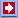Software Online Library ContactsSoftware: Business&GamesCalculator Download Analyzer Mail NavigatorOnline: Stat Calc Y(X) Finder 2D Plotter 3D Plotter Science CalcLibrary: Data Mining Statistics Machine Learning Genetic Algorithms Neuronets Fuzzy logic ForecastLinksContactsOnline -> 2D Plotter

 QuData 2D Plotter This application allows you to plot up to 3 function graphs. Enter the function in one of the text fields below the plot and press to draw it. The first function is plotted in red, the second one - in blue and the third - in green. Your functions can contain: Numbers the decimal part of the number must be separated by a dot. Operations. Following operations are permitted: "+" - add "-" - subtract "*" - multiply "/" - divide "^" - power Functions. Function consists of a name and a set of arguments in parentheses. Arguments are separated by comma (if a function has more than one argument). The following standard functions are available: "sin(x)" - sine; "cos(x)" - cosine; "tan(x)" - tangent; "ctg(x)" - cotangent; "asin(x)" - arc sine; "acos(x)" - arc cosine; "atan(x)" - arc tangent; "sinh(x)" - hyperbolic sine; "cosh(x)" - hyperbolic cosine; "tanh(x)" - hyperbolic tanhent; "exp(x)" - ex where e = 2.718...; "ln(x)" - returns the natural logarithm (base e) of x; "log(x)" - returns the logarithm (base 10) of x; "abs(x)" - absolute value of x; "sqrt(x)" - square root of x (x0.5); "pow(x, y)" - returns x raised to the power y; "max(x, y)" - returns maximum value from x and y; "min(x, y)" - returns minimum value from x and y; "random(d)" - returns uniformly distributed random number greater than 0.0 and less than 1.0, for any d; "fact(x)" - returns factorial of x (x is converted into integer); "rint(x)" - returns the integer value to closest to x; "ceil(x)" - returns the closest integer value greater than x; "floor(x)" - returns the closest integer value less than x; Variable: Only the variable named x is available You can also enter a set of empirical X and Y values into corresponding fields on the right of the plot. Each number must occupy only one string. Be sure the x and y coordinates are set in correct sequence. You can enter up to 100 000 pairs of values here. Then click the button "Plot" to plot this data. Button "Clear" will clear the data field. In the bottom right corner of the window you can change the plot range. To expand/narrow both X and Y range at once on 10%, use the "mouse" left/right button, clicking inside the plot field. Drop and drag the left "mouse" button to shift the plot region of view without scaling.